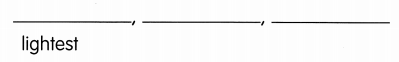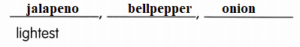Practice the problems of Math in Focus Grade 1 Workbook Answer Key Chapter 10 Weight to score better marks in the exam.

Look at the pictures.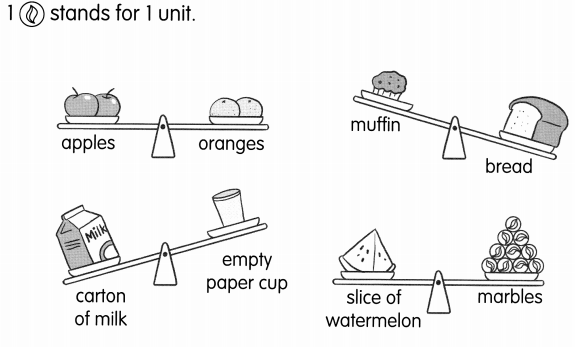Question 1.
The ___ are as heavy as the ____
Answer: The apples are as heavy as the oranges

Question 2.
The ___ is heavier than the ____

Question 3.
The ___ is lighter than the ____
Answer: The carton of milk is lighter than the empty paper cup

Question 4.
The slice of watermelon is ____ units.
Answer: The slice of watermelon is 10 units.

Chgllenaing Practice

Solve.

Question 1.
Box A has a weight of 6 beads. Box B is 2 beads heavier than Box A. Draw the beads for Box A and Box B.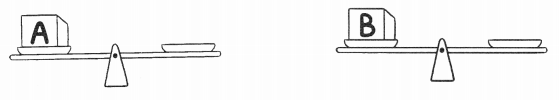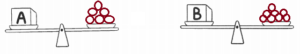Look at the pictures. Then answer the question.

Question 2.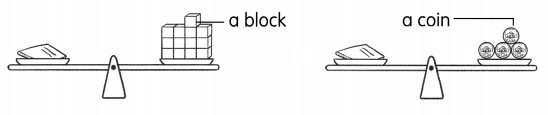Which is heavier, a block or a coin? _______
Answer: A block is heavier than a coin
Because the weight of books is equal to 13 blocks
And also the weight of books is equal to 5 coins
13 is more than 5 so blocks is heavier than coin

Write bananas or watermelon in the blanks.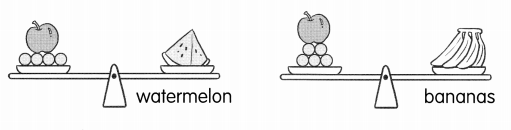Question 3.
The ____ are heavier than the ____
Answer: The bananas are heavier than the watermelons

Problem solving

Look at the plants.

Question 1.
Arrange the plants in order of their weight from the heaviest to the lightest.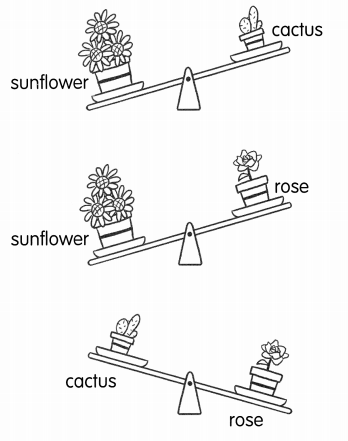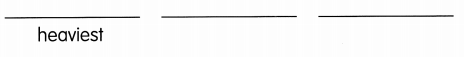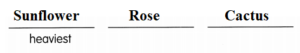Chapter Review/Test
Vocabulary
Choose the correct word.

Question 1.
The _____________ of a thing is how heavy or light ills.
Answer:  The weight of a thing is how heavy or light ills.

Question 2.
A _____________ is the quantity used for measuring a thing.
Answer: A Unit is the quantity used for measuring a thing.

Question 3.
A lion is a _____________ animal.
Answer: A lion is a wild animal.

Question 4.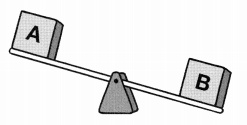Bag A is ___ than Bag B.
Answer: Bag A is lighter than Bag B.

Question 5.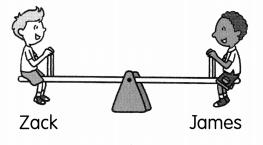James is ___ zack.
Answer: James is as heavy as zack.

Concepts and Skills

Fill in the blanks

Question 6.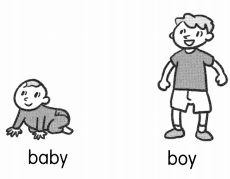The ___ is heavier
The ___ is lighter.
The baby is lighter.

Check (✓) the heavier thing.

Question 7.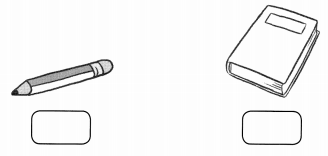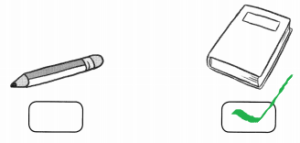Question 8.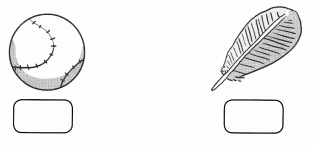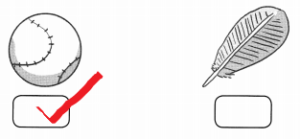Question 9.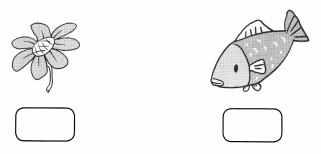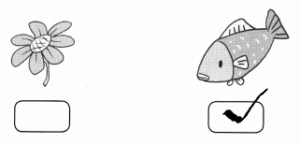Question 10.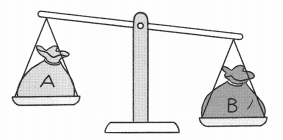Bag A is ____ than Bag B.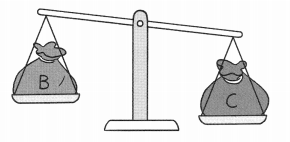Bag B is ___ than Bag C.
So, Bag A is ____ than Bag C.
Bag A is lighter than Bag B.
Bag B is lighter than Bag C.
So, Bag A is lighter than Bag C.

Question 11.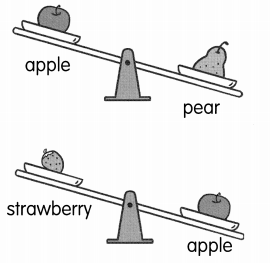The _____ is the heaviest.
The _____ is the lightest.
The pear is the heaviest.
The strawberry is the lightest.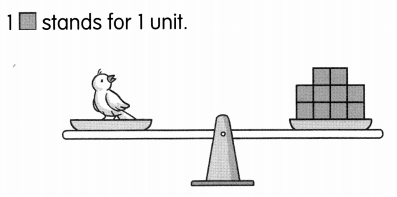Question 12.
The weight of the bird is about ___ units.
The weight of the bird is about 5 units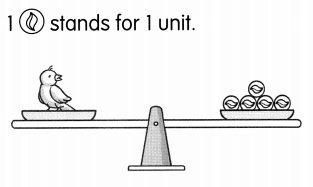.

Question 13.
The weight of the same bird is about  _____ units.

Question 14.
The number of units is different because _______
Answer: The number of units is different because there are 5 more blocks than coins

Problem Solving

Solve.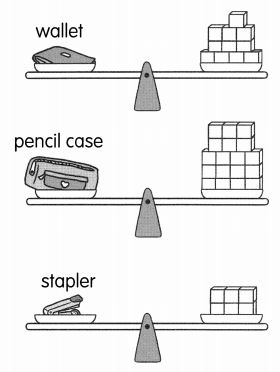Question 15.
The weight of the wallet is ________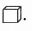Answer: The weight of the wallet is 11 unitsQuestion 16.
The weight of the pencil case is ________Answer: The weight of the pencil case is 18 unitsQuestion 17.
The weight of the stapler ¡s ________Answer: The weight of the stapler ¡s  6 unitsQuestion 18.
Which ¡s the heaviest? ______________
Answer: The pencil case is the heaviest

Question 19.
Which is the lightest? _______
Answer: The Stapler is the lightest

Question 20.
The _____________ is heavier than the ___________
Answer: The pencil case is heavier than the wallet

Question 21.
The _____________ is lighter than the _________
Answer: The stapler is lighter than the wallet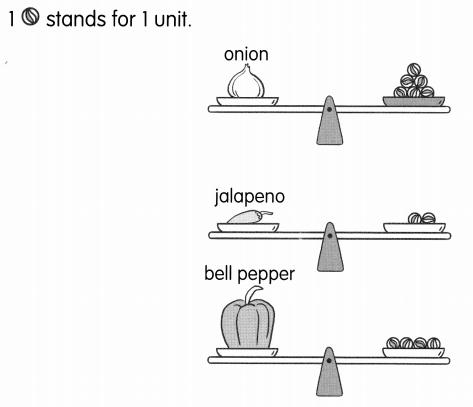Question 22.
What is the weight of the onion? ____ units
Answer: The weight of the onion is 6 units

Question 23.
What is the weight of the jalapeno? ___ units
Answer: The weight of the jalapeno is 2 units

Question 24.
What is the weight of the bell pepper? ___ units
Answer: The weight of the bell pepper is 4 units

Question 25.
Which is the heaviest? ___